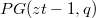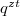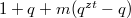﻿

### The non-existence of desarguesian$t$-parallelisms,$t$ an odd prime

#### Abstract

In this article, it is shown that when$t$ is a prime, partial Desarguesian$%t$-parallelisms in$PG(zt-1,q)$ of$m$$t$-spreads are equivalent totranslation nets of order$q^{zt}$ and degree$1+q+m(q^{zt}-q)$. Thus, abound is established for the \ number of$t$-spreads in partial Desarguesian$t$-parallelisms, when$t$ is an odd prime. This also shows that therecannot be Desarguesian$t$-parallelisms when$t$ is an odd prime.

DOI Code: 10.1285/i15900932v30n1p13

Keywords:
t-parallelism; translation planes; rational Pappian partial spreads

Classification: 51E20; 51A40

Full Text: PDFThis work is licensed under a Creative Commons Attribuzione - Non commerciale - Non opere derivate 3.0 Italia License.